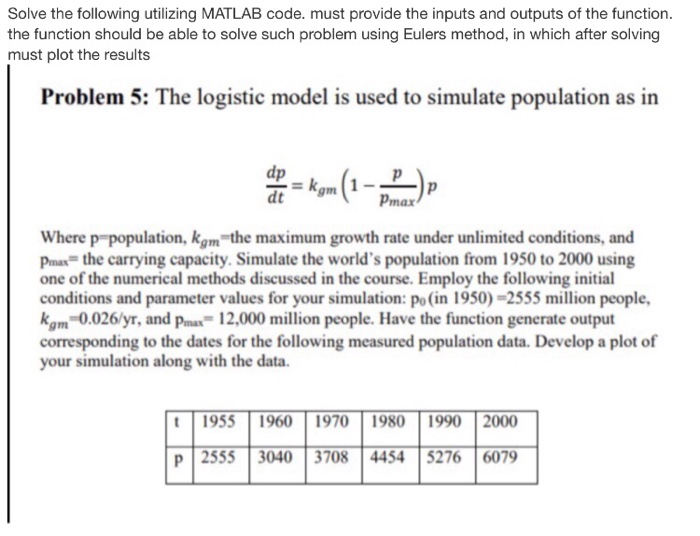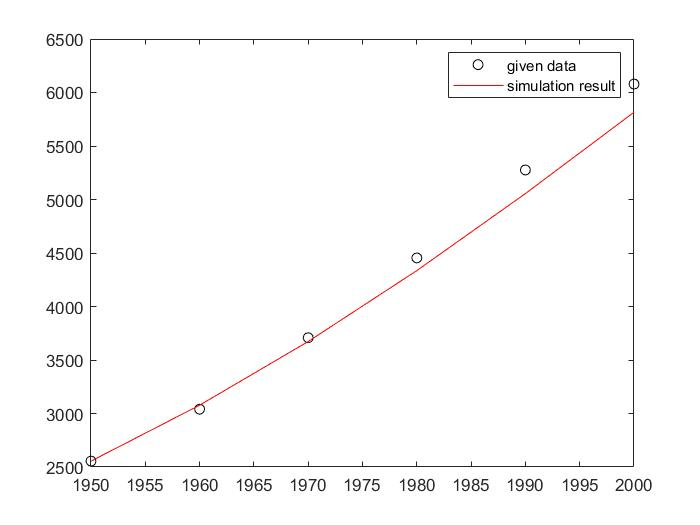Homework Help Question & Answers

Solve the following utilizing MATLAB code. must provide the inputs and outputs of the function. the function should be able to solve such problem using Eulers method, in which after solving must p...Solve the following utilizing MATLAB code. must provide the inputs and outputs of the function. the function should be able to solve such problem using Eulers method, in which after solving must plot the results Problem 5: The logistic model is used to simulate population as in Pmax Where p population, kgm the maximum growth rate under unlimited conditions, and рта? the carrying capacity. Simulate the world's population from 1950 to 2000 using one of the numerical methods discussed in the course. Employ the following initial conditions and parameter values for your simulation: po (in 1950)-2555 million people, kgm1 0.026/yr, and Prax= 12.000 million people. Have the function generte output corresponding to the dates for the following measured population data. Develop a plot of your simulation along with the data. t1955 1960 1970 1980 1990 2000 p 25553040 3708 4454 5276 6079

Code: populationestimator.m

clc
clear

t0 = 1950;
p0 = 2555;
tEnd = 2000;
h = 10;
N  = (tEnd - t0)/h;
%% Initializing solution
t = [t0:h:tEnd]';
p = zeros(N + 1, 1);
p(1) = p0;

%% Ploting given data
pg = [2555 3040 3708 4454 5276 6079];
plot(t, pg, 'ko');
hold on
%% Solving using Euler's Explicit Method
for i = 1:N
fi = funp(t(i), p(i));
p(i + 1) = p(i) + h*fi;
end

plot(t, p, 'r-');
legend('given data', 'simulation result');

Code: funp.m

function dp = funp(t, p)
kgm = 0.026;
pmax = 12000;
dp = kgm * (1 - p/pmax)*p;

end

Output:Add Answer of: Solve the following utilizing MATLAB code. must provide the inputs and outputs of the function. the function should be able to solve such problem using Eulers method, in which after solving must p...
More Homework Help Questions Additional questions in this topic.

• Use Matlab 2. Write a matlab code for fixed point iteration to find appr Use this method to solve ar323: Hint: f() (3023)l 2. Write a matlab code for fixed point iteration to find appr Use th...

Need Online Homework Help?

Get FREE EXPERT Answers
WITHIN MINUTES
Related Questions